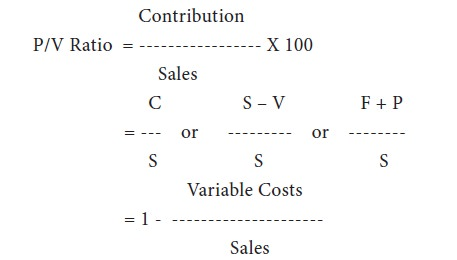Home | ARTS | Accounting For Managers | Various Elements Of Marginal Costing

# Various Elements Of Marginal Costing

Posted On :  26.02.2018 08:06 am

Various Elements Of Marginal Costing

According to the institute of cost and management accountants (icma), london, marginal cost is `the amount at any given volume of output by which aggregate costs are changed if the volume of output is increased or decreased by one unit’. Thus marginal cost is the added cost of an extra unit of output.
Mc = Direct Material + Direct Labour + Other Variable Costs
= Total Cost – Fixed Cost.
Contribution
The difference between selling price and variable cost (or marginal cost) is known as `contribution’ or `gross margin’. It may be considered as some sort of fund from out of which all fixed costs are met. The difference between contribution and fixed cost represents either profit or loss, as the case may be. Contribution is calculated thus:
Contribution = Selling Price – Variable Cost
= Fixed Cost + Profit Or – Loss
It is clear from the above equation that profit arises only when contribution exceeds fixed costs. In other terms, the point of ‘no profit no loss’ will be at a level where contribution is equal to fixed costs.
Marginal cost equation
The algebraic expression of contribution is known as marginal cost equation. It can be expressed thus:

S – V = F + P
S – V = C
C = F + P And In Case Of Loss
C = F – L
Where: S = Sales
V = Variable Cost
C = Contribution
F = Fixed Cost
P = Profit
L = Loss
Profit Volume Ratio (P/V Ratio)
The profitability of business operations can be found out by calculating the p/v ratio. It shows the relationship between contribution and sales and is usually expressed in percentage. It is also known as `marginal-income ratio’, `contribution-sales ratio’ or `variable-profit ratio’. P/v ratio thus is the ratio of contribution to sales, and is calculated thus:The ratio can also be shown by comparing the change in contribution to change in sales, or change in profit to change in sales. Any increase in contribution, obviously, would mean increase in profit, as fixed expenses are assumed to be constant at all levels of production.
Change In Contribution
P/V Ratio = -------------------------------
Change In Sales

Change In Profit
= ------------------------
Change In Sales

The importance of p/v ratio lies in its use for evaluating the profitability of alternative products, proposals or schemes. A higher ratio shows greater profitability. Management should, therefore, try to increase p/v ratio by widening the gap between the selling price and the variable costs. This can be achieved by increasing sale price, reducing variable costs or switching over to more profitable products.

Tags : Accounting For Managers - Management Accounting-Marginal Costing
Last 30 days 2142 views